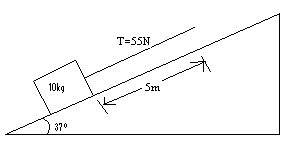# Energy conservation and string tension problem

## Homework Statement

A string exerts a tension force of 55N on a 10kg block as it moves a distance of 5m up a frictionless incline. The string pulls parallel to the incline.A) Find the total external work done on the block-earth system as the block moves 5m up the incline.

B)Find the change in gravitational potential energy for the block-earth system as the block moves 5m up the incline.

C)Find the change in kinetic energy for the block-earth system as the block moves 5m up the incline.

## Homework Equations

$$KE=\frac{1}{2}mv^{2}$$
$$PE_{g}=mgh$$
Our teacher tells us to round gravity up to 10m/s/s.

## The Attempt at a Solution

A)The external force should be the tension, so:
$$(55N)(5m) = 275J$$

B)I used trig (3-4-5 triangle) to find the height to go up 3m.
$$mgh_{f}-mgh_{i}=\Delta KE$$

$$(10)(10)(3)-(10)(10)(0)=300J$$

C)$$KE_{f}-KE_{i}=\DeltaKE$$
I am not sure what to do here because I don't know how to solve for velocity.

kuruman
Homework Helper
Gold Member
2021 Award
What does the work-energy theorem say about change in kinetic energy?

What does the work-energy theorem say about change in kinetic energy?

$$W_{net}=KE_{f}-KE_{i}$$

If this is right, then I can set 275J equal to

$$KE_{f}-KE_{i}$$

$$\frac{1}{2}mv_{f}^{2}-\frac{1}{2}mv_{i}^{2}=275J$$

$$5v_{f}^{2}-5v_{i}^{2}=275J$$

But then I will have two variables $$v_{f}$$ and $$v_{i}$$

I think I am on the wrong track.

kuruman
Homework Helper
Gold Member
2021 Award
$$W_{net}=KE_{f}-KE_{i}$$
This is right.
If this is right, then I can set 275J equal to

$$KE_{f}-KE_{i}$$
No, you cannot. Tension is not the only force that does work. What other force is there?

$$\frac{1}{2}mv_{f}^{2}-\frac{1}{2}mv_{i}^{2}=275J$$

$$5v_{f}^{2}-5v_{i}^{2}=275J$$

But then I will have two variables $$v_{f}$$ and $$v_{i}$$
You don't care about the initial and final speeds. The problem is asking you to find the change in kinetic energy, not the initial and final speeds. If you find the correct Wnet, that is the change in kinetic energy.

Tension is not the only force that does work.

It asks what the change for the block-earth system is. On a free body diagram for the block, I have three forces:

I have the Normal force (80N) by the ramp on the block,
the tension force (55N) ,
and a weight force (80N in y-dir, 60N in x-dir). (no friction)

Is the other force doing work the Normal force?

If so, then I am not sure how to calculate the work because the distance is perpendicular.

kuruman
Homework Helper
Gold Member
2021 Award
Yes there are three forces. As you say, the normal force does zero work. So what is the net work, i.e. the total work done by all three forces?

So what is the net work, i.e. the total work done by all three forces?
This is where I am getting confused.
The problem is defining the system as block-earth, so if I am not mistaken, the weight force is the only force not doing work on the system.
That would leave Normal and Tension forces.
Normal is perpendicular, so equal to zero,
Tension is 275J,
so total work is [275+0] 275 Joules.
Tension is not the only force that does work. What other force is there?
You said earlier I can't set the equations equal to 275J because there are other forces. What am I missing here?
Do I need to add in the weight force? I think it should be included in the block-earth system, not as external work.

kuruman